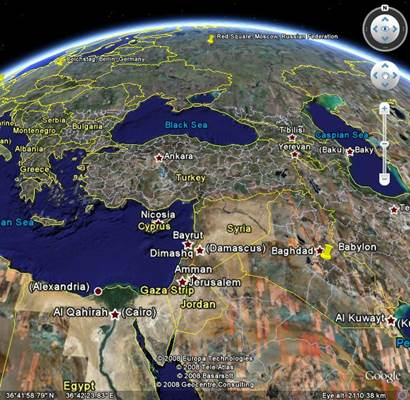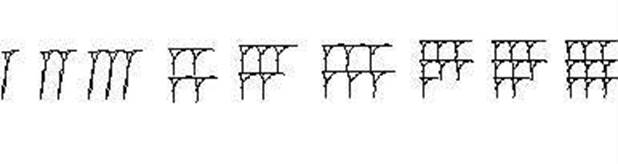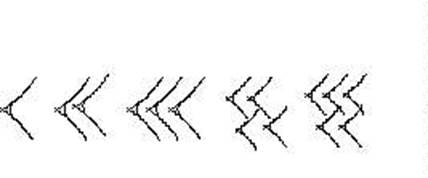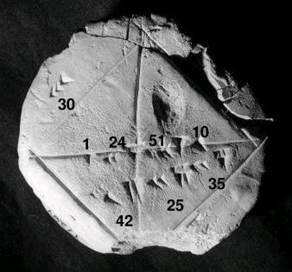# Counting in Babylon

Michael Fowler, UVa Physics

## The Earliest Written LanguageSumer and Babylonia, located in present-day Iraq, were probably the first peoples to have a written language, beginning in Sumer in about 3100 BC.  The language continued to be written until the time of Christ, but then it was completely forgotten, even the name Sumer became unknown until the nineteenth century.

From the earliest times, the language was used for business and administrative documents.  Later, it was used for writing down epics, myths, etc., which had earlier probably been handed down by oral tradition, such as the Epic of Gilgamesh.

## Weights and Measures: 60s everywhere!

In about 2500 BC, by Royal Edict, weights and measures were standardized in Babylon.  This was a practical business decision, which without doubt eliminated much tension in the marketplace.

The smallest unit of weight was the grain (about 45 milligrams).  What use was that?  At first, the currency was in fact barleycorn!  (They later moved to silver and gold ingots.)  The shekel was 180 grains (about ¼ ounce), the mina 60 shekels, and the talent 3600 shekels (about 67 pounds).

 1 talent = 60 minas= 3600 shekels= approx. 60 lbs. 1 mina= 60 shekels= approx. 1 lb. 1 shekel= 180 grains= approx. 0.25oz. 1 grain= approx. 45 mg.

The smallest unit of length was--surprise--the barleycorn, called she, about 1/10 inch.

Next came the finger, or shu-si, equal to 6 she, about 2/3 of an inch

The cubit (or kush) was 30 fingers, about 20 inches

The nindan (or GAR, or rod) was 12 cubits, 20 feet or 6 meters.

The cord or rope (used in surveying) was 120 cubits, 200 feet, that is, 3600 fingers.

The league (also called stage and beru) was 180 cords, about seven miles.

The basic unit of area was the sar, one square nindan, 400 sq ft, a garden plot.

The gin was 1/60 sar.

By 2000 BC, there was a calendar with a year of 360 days, 12 months of 30 days each, with an extra month thrown in every six years or so to keep synchronized with astronomical observations.  (According to Dampier, A History of Science, Cambridge, page 3, the day was divided into hours, minutes and seconds, and the sundial invented.  He implies this is about 2000 BC.  He doesn't say how many hours in a day, and Neugebauer (The Exact Sciences in Antiquity, Dover, page 86) claims the Egyptians were the first to come up with twenty-four.)

The circle was divided into 360 degrees.

Notice that all these standards of measurement include multiples of 60 frequently--obviously, 60 was the Babylonians' favorite number.

## Number Systems: Ours, the Roman and the Babylonian

To appreciate what constitutes a good counting system, it is worthwhile reviewing briefly our own system and that of the Romans.  The Roman system is in a way more primitive than ours:  X always means 10, C means 100 and I means 1.  (You might be thinking:  this isn't quite true--they reversed numbers to indicate subtraction, such as IV for 4.  In fact it appears they didn't, they used IIII, and IV is more recent.  There's an article on all this in Wikipedia.)

By contrast, in our system 1 can mean 1 or 10 or 100 depending on where it appears in the expression--the 1 in 41 means a different quantity from the 1 in 145, for example.  We say the value of a symbol has "positional dependence"--its actual value depends on where in the expression it appears.  Our convention, as you well know, is that the number to the far right in our system is the number of 1's, the number to its immediate left is the number of 10's, to the left of that comes the number of 10×10's, then of 10×10×10's and so on.  We use the same set of symbols, 1,2,3,4,5,6,7,8,9,0 in each of these positions, so the value of such a symbol in a number depends on its position in that number.

To express quantities less than 1, we use the decimal notation.  We put a dot (in some countries a comma is used) and it is understood that the number to the immediate left of the dot is the number of 1's, that to the immediate right the number of tenths (10-1 's in mathematical notation), the next number is the number of hundredths (10-2 's) and so on.  With this convention, ½ is written .5 or 0.5 and 1/5 is .2.  Unfortunately, 1/3 becomes .33333..., rather inconveniently, and 1/6 and 1/7 similarly go on for ever.  (Actually, this decimal system with the dot is, historically speaking, rather a recent invention--it was created by a Scotsman called Napier about 400 years ago. )

To get back to comparing the Roman system with our own, notice that the Romans did not have a 0, zero.  This is why it is important to have a different symbol for ten and one, X and I are easily distinguished.  If we didn't have a zero, one and ten would both be represented by 1, although we might be able to distinguish them in a column of figures by placing them in different columns.

After those preliminary remarks, we are ready to look at the Babylonian system.  It's written on clay tablets – that's why we still have original copies around!

Their number system has only two basic elements, the first of which is clear on examining the first nine numbers:Evidently, these nine numbers are all constructed of a single element, a mark easily gouged with one twist of a stick in the soft clay, and the number of times this element is repeated is the number represented.  The sticks used to make the marks were wedge shaped, the numbers 10, 20, 30, 40, 50, are represented by the symbols:It is clear that again we have simple repetition of a basic element, which we will conveniently represent by <, and again it's a mark not difficult to make in the soft clay.  Thus, any number between 1 and 59 is represented by a symbol from the second diagram followed in the usual case by one from the first diagram, so 32 would be written <<<11, possibly with the <'s more elegantly arranged.

When they get to 60, the Babylonians start again in a similar way to our starting again at 10. Thus, 82 is written as 1<<11, where the first 1 represents 60.

So the Babylonian system is based on the number 60 the same way ours is based on 10.  Ours is called a "decimal" system, theirs a "sexagesimal" system.

There are some real problems with the Babylonian number system, the main one being that nobody thought of having a zero, so both sixty and one look exactly the same, that is both are represented by 1!  Actually, it's even worse--since there is no decimal point, the way to write 1/2, which we write 0.5, for five tenths, they would write <<<, for thirty sixtieths--but with no zero, of course, and no dot either.  So if we see <<< on a clay tablet, we don't know if it means 1/2, 30 or for that matter 30×60, that is, 1800.

This is in fact not as bad as it sounds--sixty is a very big factor, and it will usually be clear from the context if <<< should be interpreted as 1/2 or 30.  Also, in columns of figures, a <<< representing 30 was often put to the left of <<< representing 1/2.

## Fractions

In real life commercial transactions, simple addition and even multiplication are not that difficult in most number systems.  The hard part is division, in other words, working with fractions, and this comes up all the time when resources must be divided among several individuals.  The Babylonian system is really wonderful for fractions!

The most common fractions, 1/2, 1/3, 1/4, 1/5, 1/6 all are represented by a single number (1/2= <<< , 1/3= << , 1/5= <11, etc.).  That is, these fractions are exact numbers of sixtieths--sixty is the lowest number which exactly divides by 2, 3, 4, 5, and 6.  This is a vast improvement on the decimal system, which has infinite recurrences for 1/3 and 1/6, and even ¼ needs two figures: .25.

(Of course, even in Babylonian, eventually we are forced to go to the second "sexagesimal" number, which would be the number of sixtieths of sixtieths, that is, of three-thousand-six-hundredths.  For example, 1/8 is seven-and-a-half sixtieths, so would be written as seven followed by thirty--for seven sixtieths plus thirty sixtieths of a sixtieth.  And, 1/7 is as much of a headache as it is in our own system.)

## Ancient Math Tables: Reciprocals

In order to make their bookkeeping as painless as possible, the Babylonians had math tables: clay tablets with whole lists of reciprocals.  The reciprocal of a number is what you have to multiply it by to get 1, so the reciprocal of 2 is 1/2 written 0.5 in our system, the reciprocal of 5 is 1/5 written 0.2 and so on.

The point of having reciprocal tables is that dividing by something is the same as multiplying by the reciprocal, so using the tables you can replace division by multiplication, which is a lot easier.

Surviving clay tablet examples of Babylonian reciprocal tablets look like this:

 11 <<< 111 << 1111 <11111 11111 <11 11111111 1111111 <<<

We have cheated a bit here--the numbers 4, 5, 6, etc. in both columns should really have their 1's stacked as in the first figure above. How good is your Babylonian? Check out this table: the left-hand column is 2, 3, 4, 5, 8, the right hand column represents the corresponding fraction, ½, etc., in Babylonian.

## How Practical are Babylonian Weights and Measures?

Let's take as an example how much food a family needs.  If they consume 120 shekels of grain each day, for example, that's 12 talents of grain per year.  (One talent = 3600 shekels).  Just imagine the parallel calculation now: if the family consumes 30 ounces of grain a day, what is that in tons per year?  If you were transported to the Babylon of four thousand years ago, you would hardly miss your calculator!  (Admittedly, the Babylonian calculation is a bit more difficult every six years when they throw in an extra month.)

## Pythagoras' Theorem a Thousand Years before PythagorasSome of the clay tablets discovered contain lists of triplets of numbers, starting with (3, 4, 5) and (5, 12, 13) which are the lengths of sides of right angled triangles, obeying Pythagoras' "sums of squares" formula.  In particular, one tablet, now in the Yale Babylonian Collection, this photograph by Bill Casselman, shows a picture of a square with the diagonals marked, and the lengths of the lines are marked on the figure: the side is marked <<< meaning thirty (fingers?) long, the diagonal is marked: <<<<11 <<11111 <<<11111. This translates to 42, 25, 35, meaning 42 + 25/60 + 35/3600.  Using these figures, the ratio of the length of the diagonal to the length of the side of the square works out to be 1.414213…

Now, if we use Pythagoras' theorem, the diagonal of a square forms with two of the sides a right angled triangle, and if we take the sides to have length one, the length of the diagonal squared equals 1 + 1, so the length of the diagonal is the square root of 2.  The figure on the clay tablet is incredibly accurate--the true value is 1.414214…  Of course, this Babylonian value is far too accurate to have been found by measurement from an accurate drawing--it was clearly checked by arithmetic multiplication by itself, giving a number very close to two.